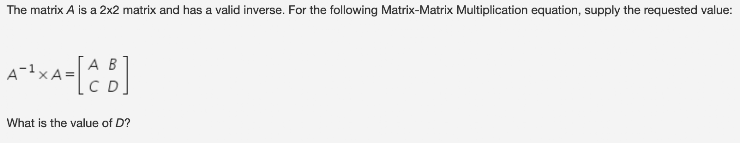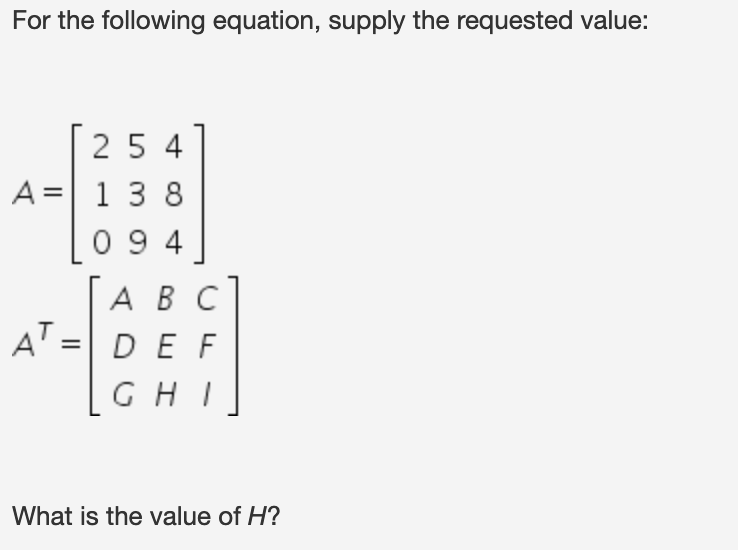Linear Algebra Assessment
Full Name *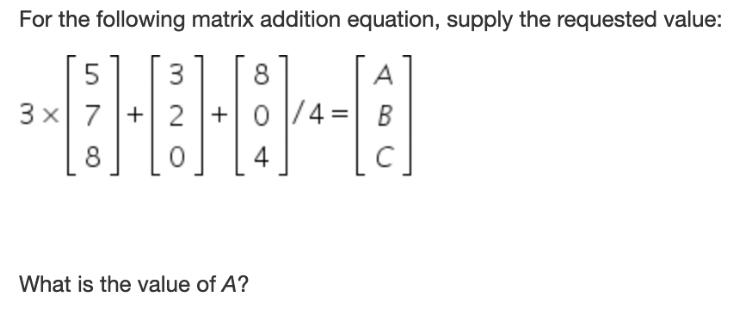Matrix-Vector Multiplication *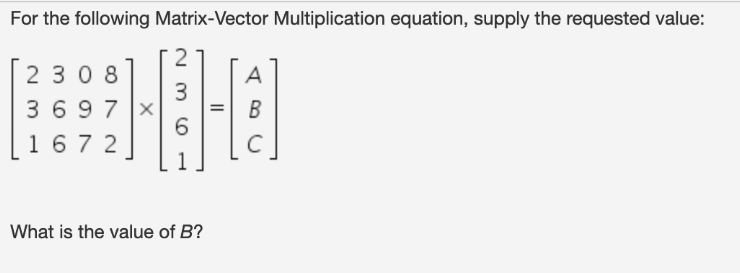Matrix-Matrix Multiplication *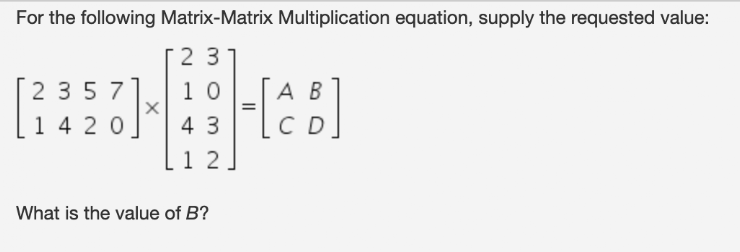Properties of Matrix Multiplication 1 *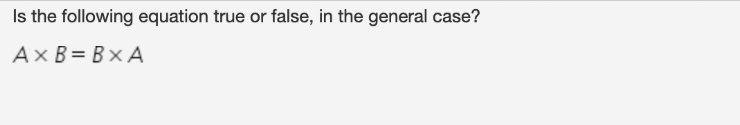Properties of Matrix Multiplication 2 *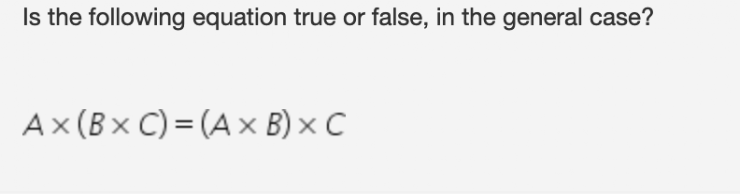Matrix Inverse *